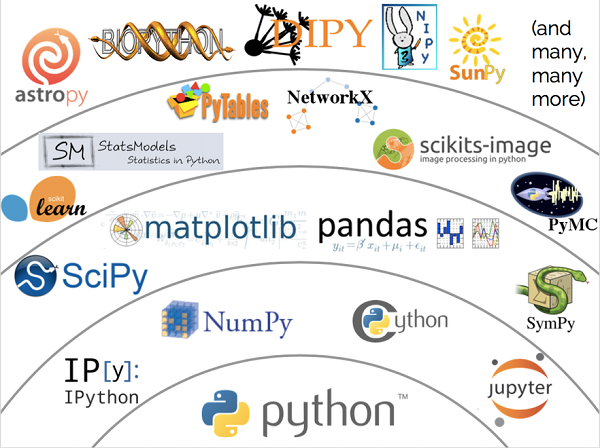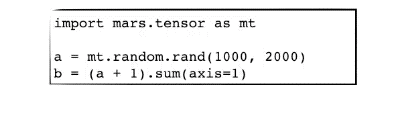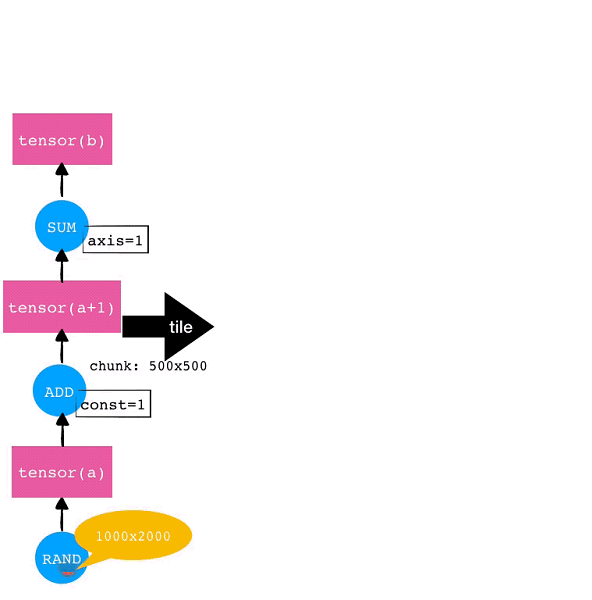# Community

Blog Events Webinars Tutorials Forum
×
Community Blog Mars – Matrix-based Universal Distributed Computing Framework

# Mars – Matrix-based Universal Distributed Computing Framework

In this article, we discuss how Mars can help researchers in the scientific computing field solve large-scale multidimensional matrix operations.

We are pleased to announce our new project, Mars, which is a matrix-based universal distributed computing framework. The open source code of Mars is already available in GitHub: https://github.com/mars-project/mars .

## Background

### Python

Python is a well-established language that continues widespread adoption in scientific computing, machine learning, and deep learning.

Hadoop, Spark, and Java are still dominant in the big data field. However, there are also a large number of PySpark users on the Spark website.

In the deep learning field, most libraries (TensorFlow, PyTorch, MXNet, and Chainer) support Python, which is the most widely used language for these libraries.

Python users are also considered as an important group in MaxCompute.

### PyData (NumPy, SciPy, Pandas, scikit-learn, matplotlib, and scikit-learn)

A variety of packages are available for Python in the data science field. The following picture shows the entire Python data science technology stack.As we can see, NumPy is the foundation and sitting on top of NumPy are libraries such as SciPy for science and engineering, Pandas for data analysis, the machine learning library scikit-learn, and matplotlib for data visualization.

In NumPy, the most important core concept is ndarray (multidimensional arrays). Libraries such as Pandas and scikit-learn are all built based on this data structure.

## Problems with Existing Computing Engines

Python continues to grow in popularity in the aforementioned fields, and the PyData technology stack provides data scientists with the computation and analysis of multidimensional matrices on DataFrame, and machine learning algorithms based on two-dimensional matrices. However, these libraries for Python are all limited to computing on a single machine. In the era of big data, these libraries cannot meet the processing capacity requirement in scenarios with very large volumes of data.

Although many SQL-based computing engines are available in the era of big data, they are not suitable for large-scale multidimensional matrix operations in the scientific computing field. On one hand, a considerable number of users, especially data scientists, have grown accustomed to mature standalone libraries. They don't want to change their habits and start to learn new libraries and syntax from scratch.

On the other hand, although ndarray/tensor is also the most basic data structure in the deep learning field, they are limited to deep learning and are not suitable for large-scale multidimensional matrix operations.

Based on these concerns, we developed Mars, a tensor-based universal distributed computing framework. In the early stage, we focused on how to make the best tensor layer.

## Detailed Analysis of Mars

The core of Mars is implemented in Python. This allows us to utilize existing achievements in the Python community. We can fully utilize libraries such as NumPy, CuPy, and Pandas as our small computing units so that we can quickly and stably build our whole system. In addition, Python itself provides easy inheritance of C/C++, and we don't have to worry about the performance of the Python language itself because we can rewrite performance hotspotting modules in C/Cython.

The next section focuses on the Mars tensor, that is, multidimensional matrix operations.

### Numpy API

One of the reasons behind the success of NumPy is its simple and easy-to-use API. The API can be directly used as our interface in the Mars tensor. Based on the NumPy API, users can flexibly write code to process data and even implement various algorithms.

The two following paragraphs of code implement a feature by using NumPy and the Mars tensor respectively.

``````import numpy as np

a = np.random.rand(1000, 2000)
(a + 1).sum(axis=1)
import mars.tensor as mt

a = mt.random.rand(1000, 2000)
(a + 1).sum(axis=1).execute()``````

The preceding example creates a 1000x2000 matrix of random numbers, adds 1 to each element and performs the sum operation on axis=1 (row).

Mars has implemented around 70% of the common NumPy interfaces.

We can see that only the "import" line is replaced. Users only need to invoke "execute" to explicitly trigger the computation. The advantage of using "execute" to explicitly trigger the computation is that we can have more optimizations of the intermediate process to efficiently perform computations.

However, the disadvantage of static graphs is the reduced flexibility and increased complexity of debugging. In the next version, we will provide the instant/eager mode to trigger computation targeting each action. This allows users to perform efficient debugging and use the Python language for cycling. However, the performance will be reduced accordingly.

### Use GPU for Computing

The Mars tensor also supports GPU computing. For interfaces created in a matrix, the `gpu=True` option is available to specify the assignment to a GPU. Subsequent computations on that matrix will be performed on the GPU.

``````import mars.tensor as mt

a = mt.random.rand(1000, 2000, gpu=True)
(a + 1).sum(axis=1).execute()``````

The preceding example assigns a to the GPU, so subsequent computations will be performed on the GPU.

### Sparse Matrix

The Mars tensor supports creating sparse matrices (only two-dimensional matrices are currently supported). For example, to create a sparse unit matrix, simply specify `sparse=True`.

``````import mars.tensor as mt

a = mt.eye(1000, sparse=True, gpu=True)
b = (a + 1).sum(axis=1)``````

The preceding example shows that the GPU and Sparse options can be specified at the same time.

## Superstructures Based on the Mars Tensor

Superstructures have not been implemented in Mars. This section describes components that we aim to build on Mars.

### DataFrame

Some of you may know the PyODPS DataFrame library, which is one of our previous projects. PyODPS DataFrame allows users to write syntax similar to Pandas syntax and perform computations on ODPS. However, due to the limits of ODPS itself, PyODPS DataFrame cannot implement all the functions of Pandas (for example, index) and has different syntax.

Based on the Mars tensor, we provide DataFrame that is 100% compatible with Pandas syntax. With Mars DataFrame, users are not limited by the memory of a single machine. This is one of our main objectives in the next version.

### Machine Learning

Some scikit-learn algorithms are entered in the form of two-dimensional NumPy ndarrays. We will also provide distributed machine learning algorithms. Roughly three methods are available for this purpose:

1. Invoke scikit-learn algorithms directly on each worker, because some scikit-learn algorithms support partial_fit.
2. Provide Mars-based Joblib backends. Because scikit-learn uses Joblib for parallelization, we can implement Joblib backends to run scikit-learn algorithms directly in the distributed Mars environment. However, this method still requires the input of NumPy ndarrays. Therefore, the total data input is still limited by memory.
3. Implement machine learning algorithms based on the Mars tensor. Despite the highest workload required, this method allows these algorithms to utilize the capabilities of the Mars tensor, for example, GPU computing. In the future, we need more of you to contribute your code and build the Mars ecology together.

## Fine-Grained Functions and Classes

The core of Mars is an actor-based fine-grained scheduling engine. Therefore, users can actually write some parallel Python functions and classes to implement fined-grained control. We may provide the following types of interfaces.

### Function

Users write common Python functions and schedule functions `by using mars.remote.spawn` to make them run on Mars in a distributed manner.

``````import mars.remote as mr

return x + y

data = [
(1, 2),
(3, 4)
]

for item in data:

`mr.spawn` allows users to easily build distributed programs. Users can also use `mr.spawn` in functions to write very fine-grained distributed executors.

### Class

Sometimes users need stateful classes to perform actions like updating status. These classes are called RemoteClasses in Mars.

``````import mars.remote as mr

class Counter(mr.RemoteClass):
def __init__(self):
self.value = 0

def inc(self, n=1):
self.value += n

counter = mr.spawn(Counter)
counter.inc()``````

Currently these functions and classes have not been implemented and are just in the conceptual stage. Therefore, the preceding interfaces are subject to changes.

## Internal Implementation

In this section we will briefly show the internal principles of the Mars tensor.

### Client

No actual computations are performed on the client. When a user writes code, we only record that user's operations in memory by using graphs.

The Mars tensor has two important concepts: operands and tensors (indicated by the blue circles and pink cubes respectively in the following diagram). An operand represents an operator and a tensor represents a generated multidimensional array.

For example, as shown in the following diagram, when a user writes the following code, we will generate corresponding operands and tensors on the graph in order.When that user explicitly invokes `"execute"`, we will submit the graph to the distributed execution environment in Mars.

Our client has no dependency on languages and only requires the same tensor graph serialization. Therefore, implementation in any language is supported. Depending on users' needs, we will determine whether we are going to provide the Mars tensor for Java in the next version.

### Distributed Execution Environment

Mars is essentially an execution and scheduling system for fine-grained graphs.

The Mars tensor receives tensor-level graphs (coarse-grained graphs) and tries to transform them into chunk-level graphs (fine-grained graphs). During the execution process, the memory should be able to store each chunk and its input. We call this process Tile.When a fine-grained chunk-level graph is received, the operands on that graph will be assigned to individual workers for execution.

## Summary

Mars was first released in September 2018 and is made fully open source now. Currently the open source code is available on GitHub: https://github.com/mars-project/mars We don't just publish our codes; our project is operating in a fully open-source manner.

We hope that more and more people can join the Mars community and build Mars together!

0 0 0
Share on

# Related Products

• ##Quick Starts

Deploy custom Alibaba Cloud solutions for business-critical scenarios with Quick Start templates.

• ##Omnichannel Data Mid-End Solution

This all-in-one omnichannel data solution helps brand merchants formulate brand strategies, monitor brand operation, and increase customer base.

• ##ApsaraDB for HBase

ApsaraDB for HBase is a NoSQL database engine that is highly optimized and 100% compatible with the community edition of HBase.

• ##MaxCompute

Conduct large-scale data warehousing with MaxCompute